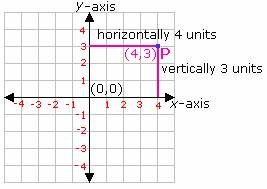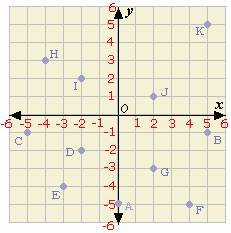Definition Of Cartesian Plane

A Cartesian Plane is a plane consisting of a set of two lines intersecting each other at right angles. The horizontal line is the X-axis and the vertical one is the Y-axis, and the point of their intersection is called the origin with the coordinates (0, 0).

The location of any point on the coordinate plane is denoted by using pair of coordinates (X, Y), where X is the horizontal distance from the origin and Y is the vertical distance from the origin.
The X and Y-axis on the coordinate plane is always perpendicular to each other.

Example of Cartesian PlaneThe location of point P is (4, 3), as the point P is placed 4 units (away from the origin) horizontally and 3 units (away from the origin) vertically.

Solved Example on Cartesian Plane

Ques: Identify the coordinates of the point C on the graph.A. (- 5, 1)
B. (5, - 1)
C. (- 1, - 5)
D. (- 5, - 1)